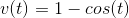## Test Prep Plan - Take a practice test

Take this practice test to check your existing knowledge of the course material. We'll review your answers and create a Test Prep Plan for you based on your results.
How Test Prep Plans work
1
2Based on your results, we'll create a customized Test Prep Plan just for you!
3Study smarter
Study more effectively: skip concepts you already know and focus on what you still need to learn.

# AP Calculus AB & BC: Help and Review Final Exam

Free Practice Test Instructions:

Choose your answer to the question and click 'Continue' to see how you did. Then click 'Next Question' to answer the next question. When you have completed the free practice test, click 'View Results' to see your results. Good luck!

#### Question 1 1. The graph shown is an example of a function that: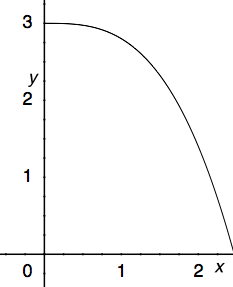#### Question 2 2. Find the derivative of f(x).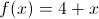#### Question 3 3. Find the domain of f(x)/g(x), if: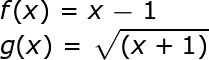#### Question 4 4. The ceiling function is graphically translated as a stepwise function. What is the limit of f(x) = ceil(x) as x approaches 4.5?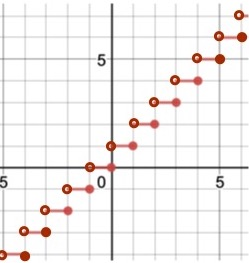#### Question 7 7. Where is f(x) differentiable?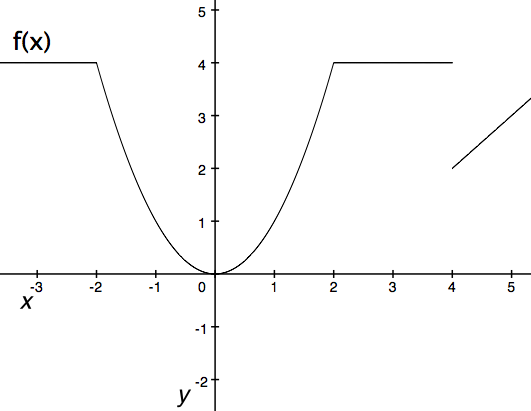#### Question 8 8. What is the equation of how L changes as a function of how x changes if h=6?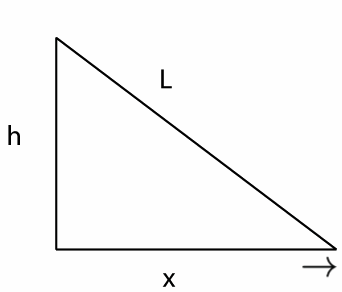#### Question 9 9. Simplify using only positive exponents.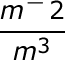#### Question 10 10. If h equals 3 m, and x is moving at a rate of 3 m / second, how fast is L changing when x equals 4m?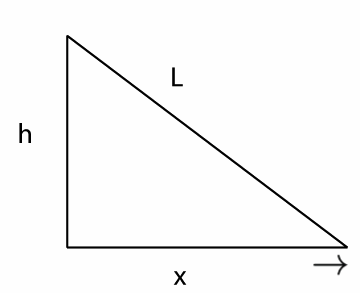#### Question 13 13. Simplify the following expression using positive exponents.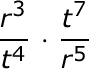#### Question 15 15. Given a velocity v(t) as defined below and a starting position of x=1 at t=0, what is the equation for your position as a function of time?